# Class IX ch 12 Herons formula module 1 ppt .pdf

28 de May de 2023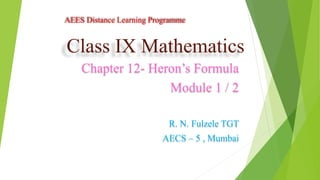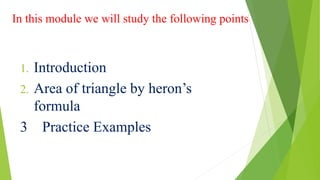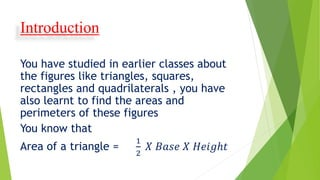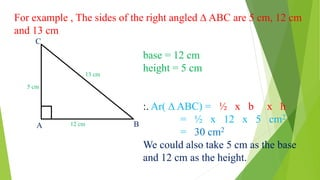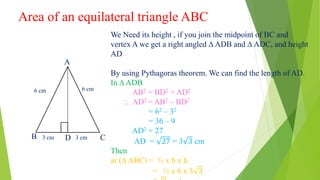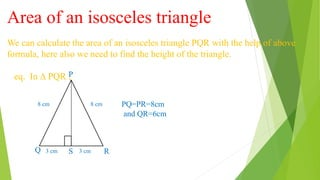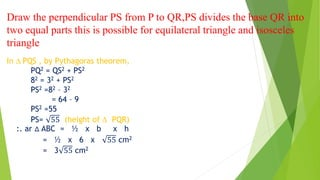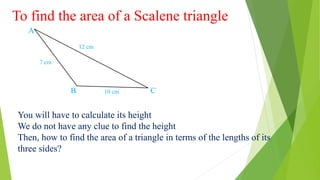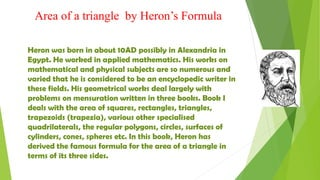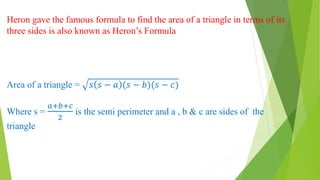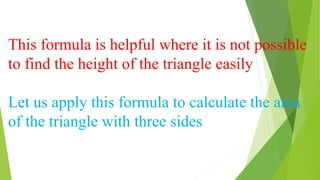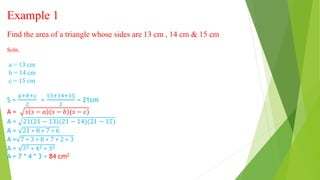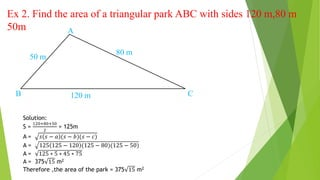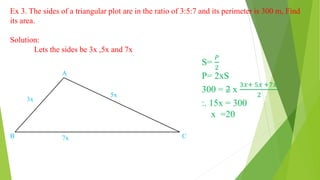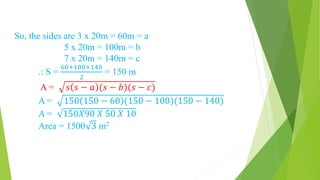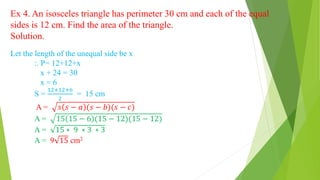1 de 17

### Class IX ch 12 Herons formula module 1 ppt .pdf

• 1. Class IX Mathematics Chapter 12- Heron’s Formula Module 1 / 2 R. N. Fulzele TGT AECS – 5 , Mumbai AEES Distance Learning Programme
• 2. In this module we will study the following points 1. Introduction 2. Area of triangle by heron’s formula 3 Practice Examples
• 3. Introduction You have studied in earlier classes about the figures like triangles, squares, rectangles and quadrilaterals , you have also learnt to find the areas and perimeters of these figures You know that Area of a triangle = 1 2 𝑋 𝐵𝑎𝑠𝑒 𝑋 𝐻𝑒𝑖𝑔ℎ𝑡
• 4. For example , The sides of the right angled ∆ ABC are 5 cm, 12 cm and 13 cm 5 cm 13 cm 12 cm C A B base = 12 cm height = 5 cm :. Ar( ∆ ABC) = ½ x b x h = ½ x 12 x 5 cm2 = 30 cm2 We could also take 5 cm as the base and 12 cm as the height.
• 5. Area of an equilateral triangle ABC C D We Need its height , if you join the midpoint of BC and vertex A we get a right angled ∆ ADB and ∆ ADC, and height AD By using Pythagoras theorem. We can find the length of AD. In ∆ ADB AB2 = BD2 + AD2 :. AD2 = AB2 – BD2 = 62 – 32 = 36 – 9 AD2 = 27 AD = 27 = 3 3 cm Then ar (∆ ABC) = ½ x b x h = ½ x 6 x 3 3 6 cm 6 cm 3 cm 3 cm A B D
• 6. Area of an isosceles triangle We can calculate the area of an isosceles triangle PQR with the help of above formula, here also we need to find the height of the triangle. eq. In ∆ PQR , 8 cm 8 cm 3 cm 3 cm Q S P R PQ=PR=8cm and QR=6cm
• 7. Draw the perpendicular PS from P to QR,PS divides the base QR into two equal parts this is possible for equilateral triangle and isosceles triangle In ∆ PQS , by Pythagoras theorem, PQ2 = QS2 + PS2 82 = 32 + PS2 PS2 =82 – 32 = 64 – 9 PS2 =55 PS= 55 (height of ∆ PQR) :. ar ∆ ABC = ½ x b x h = ½ x 6 x 55 cm2 = 3 55 cm2
• 8. To find the area of a Scalene triangle 7 cm 12 cm 10 cm B A C You will have to calculate its height We do not have any clue to find the height Then, how to find the area of a triangle in terms of the lengths of its three sides?
• 9. Area of a triangle by Heron’s Formula Heron was born in about 10AD possibly in Alexandria in Egypt. He worked in applied mathematics. His works on mathematical and physical subjects are so numerous and varied that he is considered to be an encyclopedic writer in these fields. His geometrical works deal largely with problems on mensuration written in three books. Book I deals with the area of squares, rectangles, triangles, trapezoids (trapezia), various other specialised quadrilaterals, the regular polygons, circles, surfaces of cylinders, cones, spheres etc. In this book, Heron has derived the famous formula for the area of a triangle in terms of its three sides.
• 10. Heron gave the famous formula to find the area of a triangle in terms of its three sides is also known as Heron’s Formula Area of a triangle = 𝑠 𝑠 − 𝑎 (𝑠 − 𝑏)(𝑠 − 𝑐) Where s = 𝑎+𝑏+𝑐 2 is the semi perimeter and a , b & c are sides of the triangle
• 11. This formula is helpful where it is not possible to find the height of the triangle easily Let us apply this formula to calculate the area of the triangle with three sides
• 12. Example 1 Find the area of a triangle whose sides are 13 cm , 14 cm & 15 cm Soln. a = 13 cm b = 14 cm c = 15 cm S = 𝑎+𝑏+𝑐 2 = 13+14+15 2 = 21cm A = 𝑠 𝑠 − 𝑎 (𝑠 − 𝑏)(𝑠 − 𝑐) A = 21 21 − 13 (21 − 14)(21 − 15) A = 21 ∗ 8 ∗ 7 ∗ 6 A = 7 ∗ 3 ∗ 8 ∗ 7 ∗ 2 ∗ 3 A = 72 ∗ 42 ∗ 32 A = 7 * 4 * 3 = 84 cm2
• 13. Ex 2. Find the area of a triangular park ABC with sides 120 m,80 m 50m 80 m 50 m 120 m A B C Solution: S = 120+80+50 2 = 125m A = 𝑠 𝑠 − 𝑎 (𝑠 − 𝑏)(𝑠 − 𝑐) A = 125 125 − 120 (125 − 80)(125 − 50) A = 125 ∗ 5 ∗ 45 ∗ 75 A = 375 15 m2 Therefore ,the area of the park = 375 15 m2
• 14. Ex 3. The sides of a triangular plot are in the ratio of 3:5:7 and its perimeter is 300 m, Find its area. Solution: Lets the sides be 3x ,5x and 7x 5x 3x 7x A B C S= 𝑃 2 P= 2xS 300 = 2 x 3𝑥+ 5𝑥 +7𝑥 2 :. 15x = 300 x =20
• 15. So, the sides are 3 x 20m = 60m = a 5 x 20m = 100m = b 7 x 20m = 140m = c .: S = 60+100+140 2 = 150 m A = 𝑠 𝑠 − 𝑎 (𝑠 − 𝑏)(𝑠 − 𝑐) A = 150 150 − 60 (150 − 100)(150 − 140) A = 150𝑋90 𝑋 50 𝑋 10 Area = 1500 3 m2
• 16. Ex 4. An isosceles triangle has perimeter 30 cm and each of the equal sides is 12 cm. Find the area of the triangle. Solution. Let the length of the unequal side be x :. P= 12+12+x x + 24 = 30 x = 6 S = 12+12+6 2 = 15 cm A = 𝑠 𝑠 − 𝑎 (𝑠 − 𝑏)(𝑠 − 𝑐) A = 15 15 − 6 (15 − 12)(15 − 12) A = 15 ∗ 9 ∗ 3 ∗ 3 A = 9 15 cm2